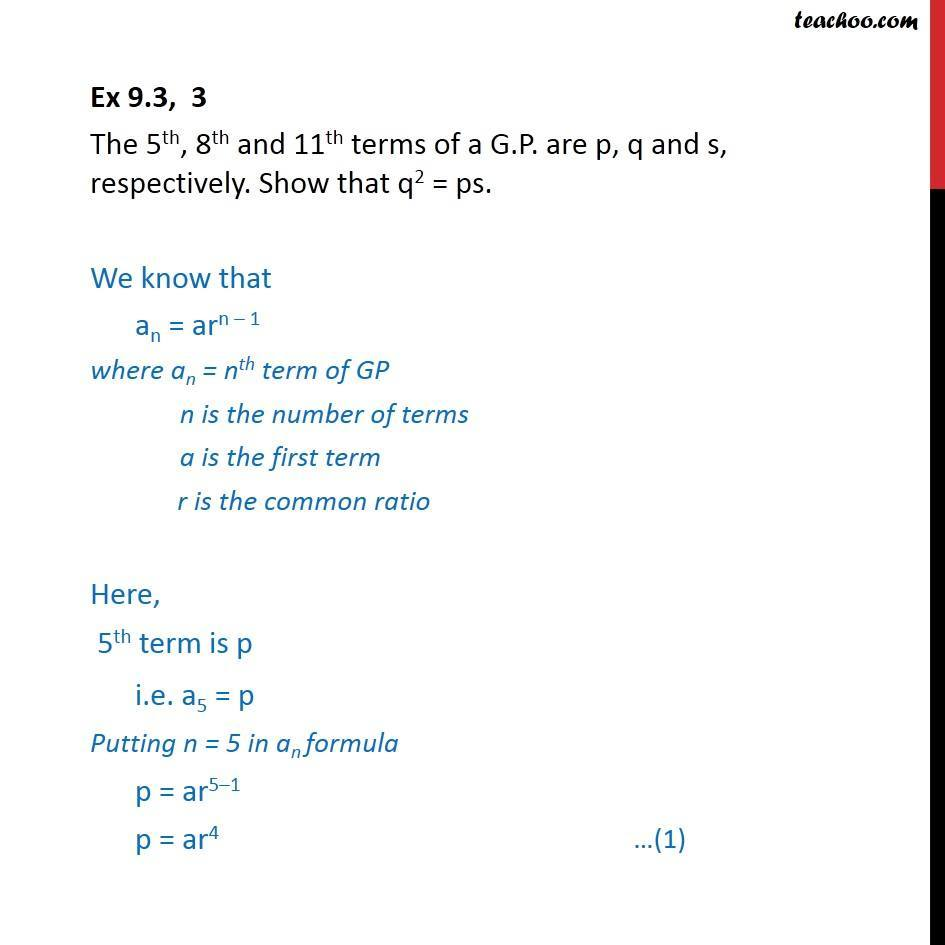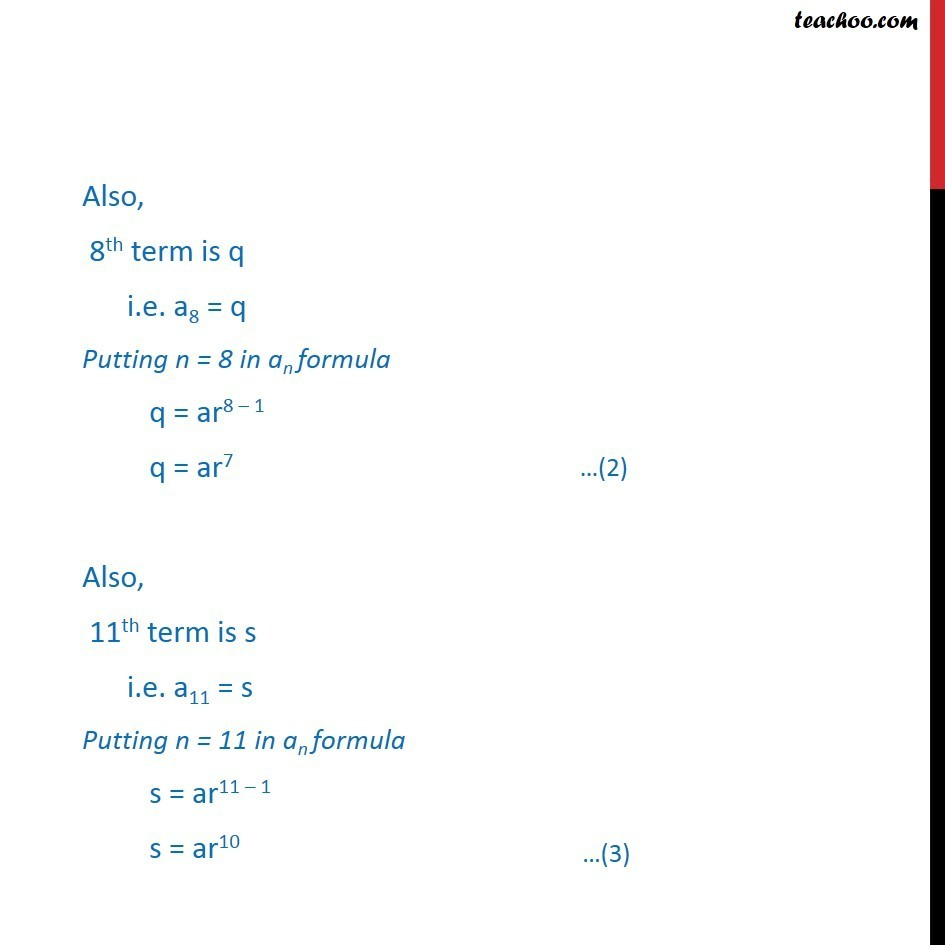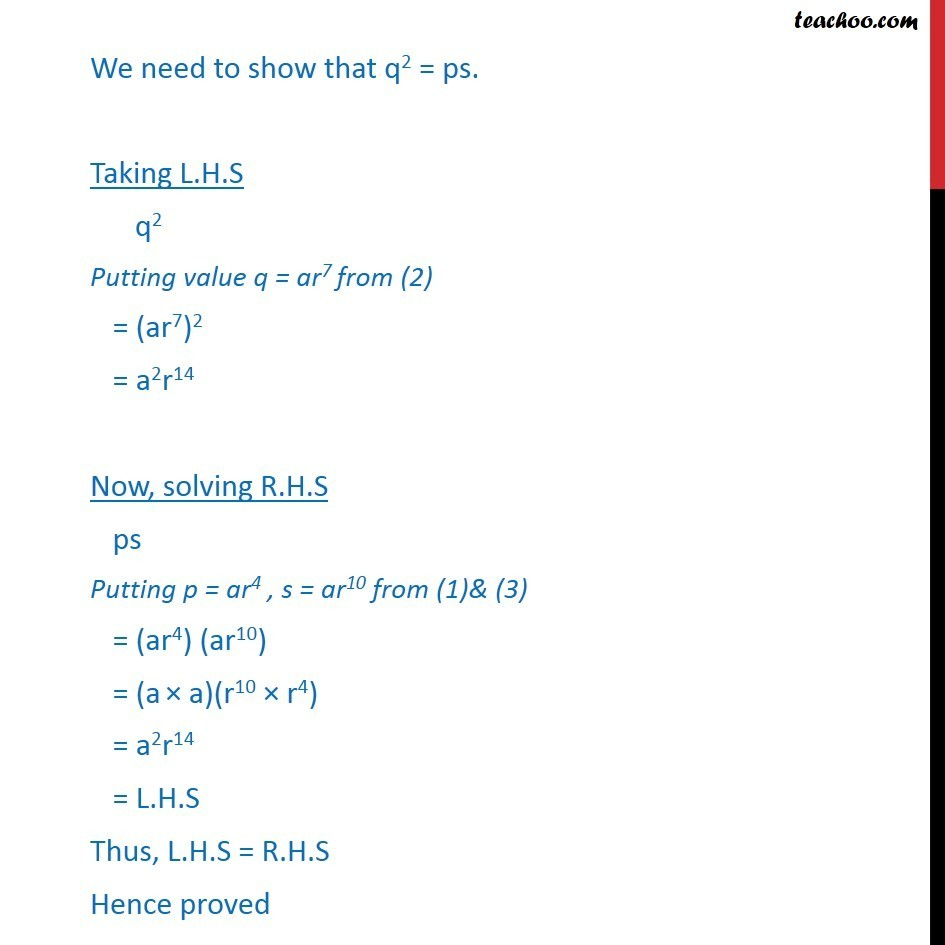Geometric Progression(GP): Formulae based

Chapter 8 Class 11 Sequences and Series
Concept wiseLearn in your speed, with individual attention - Teachoo Maths 1-on-1 Class

### Transcript

Ex 8.2, 3 The 5th, 8th and 11th terms of a G.P. are p, q and s, respectively. Show that q2 = ps. We know that an = arn – 1 where an = nth term of GP n is the number of terms a is the first term r is the common ratio Here, 5th term is p i.e. a5 = p Putting n = 5 in an formula p = ar5–1 p = ar4 Also, 8th term is q i.e. a8 = q Putting n = 8 in an formula q = ar8 – 1 q = ar7 Also, 11th term is s i.e. a11 = s Putting n = 11 in an formula s = ar11 – 1 s = ar10 We need to show that q2 = ps. Taking L.H.S q2 Putting value q = ar7 from (2) = (ar7)2 = a2r14 Now, solving R.H.S ps Putting p = ar4 , s = ar10 from (1)& (3) = (ar4) (ar10) = (a × a)(r10 × r4) = a2r14 = L.H.S Thus, L.H.S = R.H.S Hence proved